Select Page

# MCQ in English Answers for CBSE Maths 12 Science Application of Derivatives

MCQ in English Answers for CBSE Maths 12 Science Application of Derivatives to enable students to get Answers in a narrative video format for the specific question.

Expert Teacher provides MCQ Answers for CBSE Maths 12 Science Application of Derivatives through Video Answers in English language. This video solution will be useful for students to understand how to write an answer in exam in order to score more marks. This teacher uses a narrative style for a question from Application of Derivatives not only to explain the proper method of answering question, but deriving right answer too.

Please find the question below and view the Answer in a narrative video format.

Question:

## Similar Questions from CBSE, 12th Science, Maths, Application of Derivatives

Question 1 : The point on the curve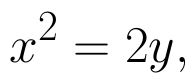which is nearest to the point (0, 5) is :

Question 2 : A balloon, which always remains spherical has a variable radius. Find the rate at which its volume is increasing with the radius when the later is 10 cm.  (View Answer Video)

Question 3 : The maximum value of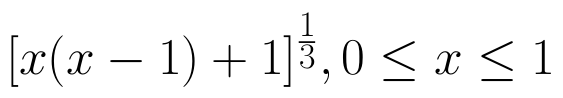is, (View Answer Video)

Question 4 : Sand is pouring from a pipe at the rate of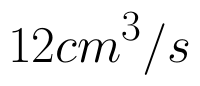. The falling sand forms a cone on the ground in such a way that the height of the cone is always one-sixth of the radius of the base. How fast is the height of the sand cone increasing, when the height is 4 cm? (View Answer Video)

Question 5 : The line y = mx + 1 is a tangent to the curve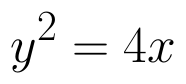if the value of m is: (View Answer Video)

### Application of Integrals

Question 1 : Find the area of the region bounded by the parabola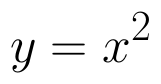and y = | x |. (View Answer Video)

Question 2 : Find the area of smaller region bounded by the ellipse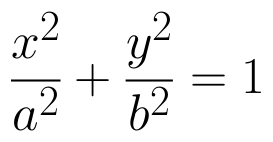and the line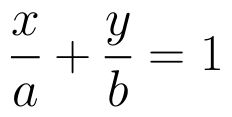. (View Answer Video)

Question 3 : Using integration find the area of region bounded by the triangle whose vertices are (-1, 0), (1, 3) and (3, 2). (View Answer Video)

Question 4 : Using the method of integration find the area bounded by the curve |x| + |y| = 1. (View Answer Video)

Question 5 : Using integration, find the area of the triangle ABC, where A is (2,3), B is (4,7) and C is (6,2). (View Answer Video)

### Relations and Functions

Question 1 : Let * be the binary operation on N given by a * b = LCM of a and b. Find 20 * 16. (View Answer Video)

Question 2 : Functions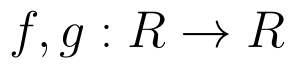are defined respectively, by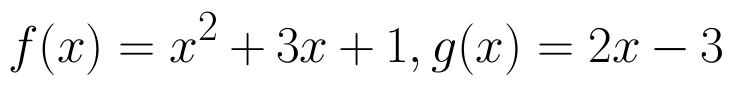, find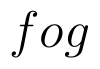. (View Answer Video)

Question 3 : A function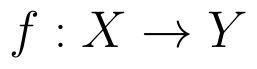is bijective if and only if, (View Answer Video)

Question 4 : A functiondefined as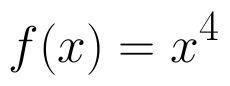is, (View Answer Video)

Question 5 : Let * be the binary operation on N given by a * b = LCM of a and b. Find 5 * 7. (View Answer Video)

### Three Dimensional Geometry

Question 1 : Find the angle between the lines whose direction ratios are a, b, c and b - c, c - a, a - b. (View Answer Video)

Question 2 : If the equation of a line AB is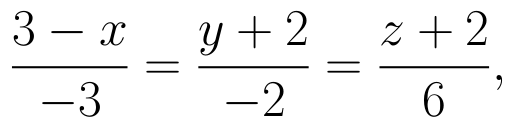then, find the direction cosines of a line parallel to AB. (View Answer Video)

Question 3 : Write the vector equation of the plane passing through the point (a, b, c) and parallel to the plane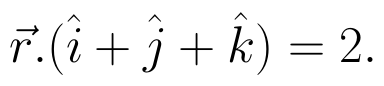(View Answer Video)

Question 4 : If the lines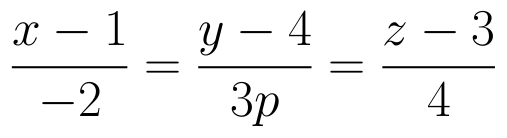and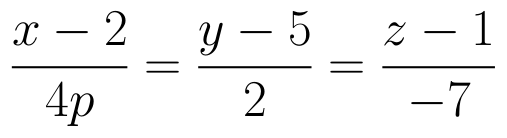are perpendicular to each other, then find the value of P. (View Answer Video)

Question 5 : If a line makes angle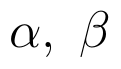and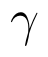with the coordinate axis, then find the value of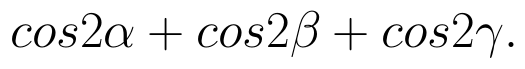(View Answer Video)